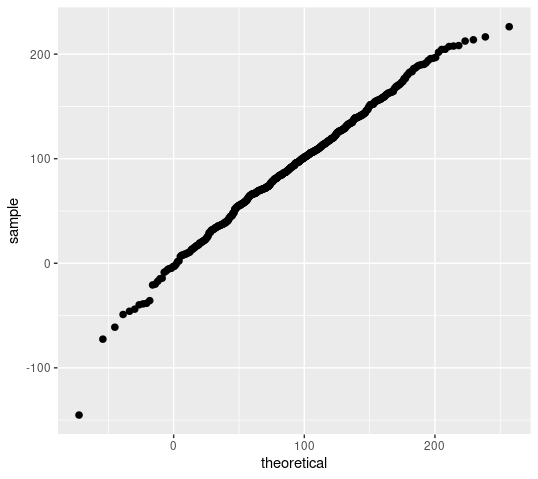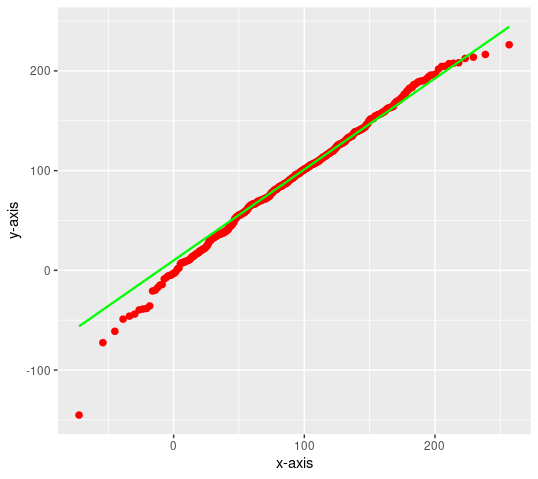Normal Probability Plot in R using ggplot2

• Last Updated : 14 Jan, 2022

A normal probability plot is a graphical representation of the data. A normal probability plot is used to check if the given data set is normally distributed or not. It is used to compare a data set with the normal distribution. If a given data set is normally distributed then it will reside in a shape like a straight line.

In this article, we are going to use ggplot2 with qqplotr to plot and check if the dataset is normally distributed using qqplot only.

Approach

• Install the following necessary libraries by pasting them in r console

install.packages(“ggplot2”)

install.packages(“qqplotr”)

• Create a random data set with a different mean and standard deviation that you want to plot.
• Plotting data using stat_qq_point() method.
• Plotting data points with line using stat_qq_line() function.

Given below is a proper implementation using the above approach

Example 1: Plotting data using stat_qq_point() method.

R

 # importing librarieslibrary(ggplot2)library(qqplotr) # creating random datarandom_values = rnorm(500, mean = 90, sd = 50) # plotting data without line and labelsggplot(mapping = aes(sample = random_values)) + stat_qq_point(size = 2)

Output:Fig. 1 Plotting Data points.

Example 2: Plotting data points with line using stat_qq_line() function.

R

 # importing librarieslibrary(ggplot2)library(qqplotr) # creating random datarandom_values = rnorm(500, mean = 90, sd = 50) # plotting data with proper labels# And adding line with proper propertiesggplot(mapping = aes(sample = random_values))+ stat_qq_point(size = 2,color = "red")+ stat_qq_line(color="green")+ xlab("x-axis") + ylab("y-axis")

Output: